Next: About this document Up: My Home Page

# Arrays and Access Formulas Lecture 20

Steven S. Skiena

One-dimensional Arrays

The easiest way to view a one - dimensional array is as a contiguous block of memory locations of length (# of array elements)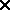(size of each element)

Because the size (in bytes) of each element is the same, the compiler can translated A into the address of the record

If A points to the first location of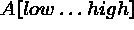of k-byte records, then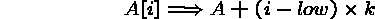This is the access formula for a one-dimensional array.

Two-Dimensional Arrays

How does the compiler know where to store element A[i,j] of a two-dimensional array? By chopping the matrix into rows, it can be stored like a one- dimensional array:

If A points to the first location of A[l1..h1,l2..h2] of k-byte records, then: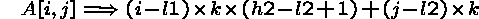Is this access formula for row-major or column-major order, assuming the first index gives the row?

For three dimensions, cut the matrix into two dimensional slabs, and use the previous formula. For k-dimensional arrays, we can find a similar formula by induction.

Thus we can access any element in a k-dimensional array in O(k) time, which is constant for any reasonably dimension.

Fortran stores its arrays in column-major order, while most other languages use row-major order. But why might we really need to know what is going on under the hood?

In C language, pointers are usually used to cruise through arrays. Cruising through a 2D array meaningfully requires knowing the order of the elements.

Also, in a computer with virtual memory or a cache, it is often faster to access elements if they are close to the last one we have read. Knowing the access function lets us choose the right way to order nested loops.

```

(*row-major*)  		 						       (*column-major*)

Do i=1 to n     						 		      Do j=1 to n

Do j=1 to n     						 		      Do i=1 to n

A[i,j] = 0      						 		      A[i,j] = 0

```

Triangular Tables

By playing with our own access functions we can build efficient arrays of whatever shape we want, including triangular and banded arrays.

Triangular tables prove useful for representing any symmetric function, such as the distance from A to B, D[a,b] = D[b,a]. Thus we can save almost half the memory of a rectangular array by storing it as a triangle

The access formula is: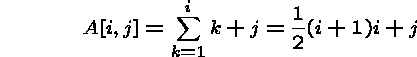since the identity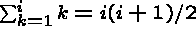can be proven by induction.

Faster than Binary Search?

Binary search takes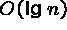time to find a particular key in a sorted array. It can be shown that, in the worst case, no faster algorithm exists. So how might we do faster?

This is not a contradiction. Suppose we wanted to search on a field containing an ID number between 1 and the number of records. Rather than doing a binary search on this field, why not use it as an index in an array!

Accessing such an array element is O(1) instead of!

Interpolation Search

Binary search is only optimal when you know nothing about your data except that it is sorted!

When you look up AAA in the telephone book, you don't start in the middle. We use our understanding of how things are named in the real world to choose where to prove next. Such an algorithm is called an interpolation search, since we are interpolating(guessing) where the key should be.

Interpolation search is only as good as our guesses. If we do not understand the data as well as you think, interpolation search can be very slow - recall the Shifflett's of Charlottesville!

With interpolation search, the cost of making a good guess might overwhelm the reduction in the number of guesses, so watch out!

The Key Ideas on Access Formulas

A pointer tells us exactly where in memory an item is.

An array reference A[i] lets us quickly calculate exactly where the ith element of A is in memory, knowing only i, the starting location of A, and the size of each array item.

Any time we can compute the exact position for an item in memory by a simple access formula, we can find it as quickly as we can compute the formula!

Must Array Indices be Integers?

We have seen that binary search is slower than table lookup. Why can't the entire world be one big array?

One reason is that many of the fields we wish to search on are not integers, for example, names in a telephone book. What address in the machine is defined by ``Skiena''?

To compute the appropriate address we need a function to map arbitrary keys to addresses. Such hash functions form the basis of an important search technique, hashing!Next: About this document Up: My Home Page

Steve Skiena
Sat Nov 1 16:09:14 EST 1997